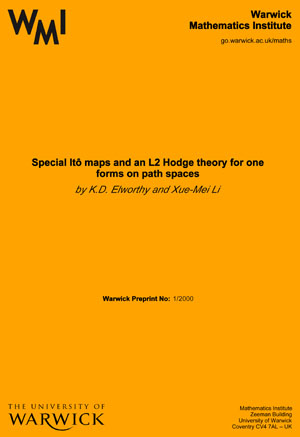# WMI Preprints 1971

Sets of constant distance from a planar set,
by M. Brown

Periodic flows on three-manifolds,
by D.B.A. Epstein

Levi factors of infinite-dimensional Lie algebras,
by I. Stewart

Pointwise periodic homeomorphisms of continua,
by N. Weaver

The Clifford algebra and the Spinor group of a Hilbert space,
by P. de la Harpe

An analytic construction of degenerating curves over complete local rings, and an analytic construction of degenerating abelian varieties over complete rings,
by D. Mumford

Tensorial extensions of central simple algebras,
by I. Stewart

Saddle points for linear differential games,
by R.J. Elliott, N.J. Kalton & L. Markus

Sylow p-subgroups and local p-solubility,
by B. Hartley

The duality pairs of wedges,
by G.J.O. Jameson

The existence of value in differential games,
by R.J. Elliott & N.J. Kalton

The existence of value in differential games of pursuit and evasion,
by R.J. Elliott & N.J. Kalton

A note on isomorphism of Markov shifts,
by M. Smorodinsky

Paracompactness: an expository essay,
by P.R. Blindell

Derivations in a class of Banach-Lie algebras,
by P. de la Harpe

Séries de Poincaré des espaces hermitiens symétriques stables,
by P. de la Harpe

Groups of diffeomorphisms and their subgroups,
by H. Omori

Permutable subgroups of infinite groups,
by S.E. Stonehewer

About interactions between Banach-Lie groups and finite dimensional manifolds,
by H. Omori and P. de la Harpe

Three notes about the cohomology of a class of Banach-Lie algebras,
by P. de la Harpe & R. Ramer

Calcul des elements primitifs dans la cohomologie des algebres de Lie classiques,
by P. de la Harpe

A geometric approach to homology theory: parts I & II,
by C.P. Rourke & B.J. Sanderson

A geometric approach to homology theory: a set of seven papers,
by S. Buoncristiano, C.P. Rourke & B.J. Sanderson

Some counterexamples in the theory of analytic subsets of Banach spaces,
by M.J. Field

A note on F-reducibility,
by B. Hartley

On the automorphism group of a 2-group,
by T.O. Hawkes

Surfaces of constant mean curvature in constant curvature manifolds,
by D.A. Hoffman

A topological classification of crystal lattices,
by R.L.E. Schwarzenberger

### Search WMI Preprint Catalogue: